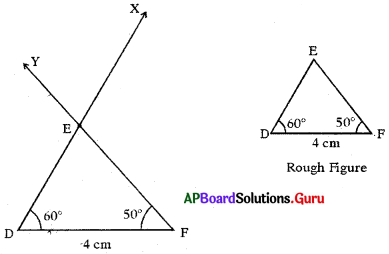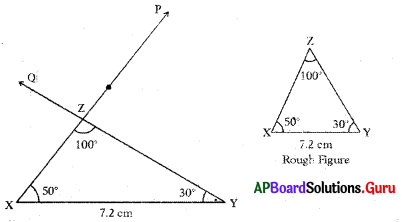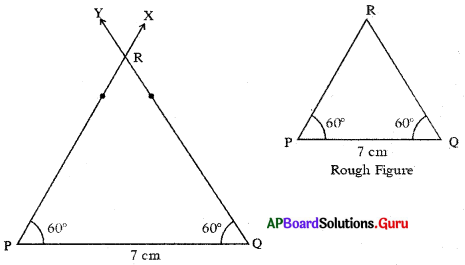# AP Board 7th Class Maths Solutions Chapter 10 Construction of Triangles Ex 10.3

SCERT AP 7th Class Maths Solutions Pdf Chapter 10 Construction of Triangles Ex 10.3 Textbook Exercise Questions and Answers.

## AP State Syllabus 7th Class Maths Solutions 10th Lesson Construction of Triangles Ex 10.3

Question 1.
Construct ∆DEF with measurements ∠D = 60°, ∠F = 50° and DF = 4 cm.
Given measurements of ∆DEF are ∠D = 60°, ∠F = 50° and DF = 4 cm.Steps of Construction :

1. Draw a rough sketch of triangle and label it with given measurements.
2. Draw a line segment with DF = 4 cm.
3. Draw a ray DX such that ∠FDX = 60°.
4. Draw another ray FY such that ∠DFY = 50° which intersects the previous ray at E. Thus, required ∆DEF is constructed with the given measurements.Question 2.
Construct the triangle with the measurements XY = 7.2 cm, ∠Y = 30° and ∠Z = 100°.
Given measurements of ∆XYZ are XY = 7.2 cm, ∠Y = 30° and ∠Z = 100°.
We know stun of angles of triangle is 180°
So, ∠X + ∠Y + ∠Z = 180°
⇒ ∠X + 30° + 100° = 180°
⇒ ∠X= 180°-130°
∴ ∠X = 50°Steps of Construction:

1. Draw a rough sketch of triangle and label it with given measurements.
2. Draw a line segment with XY = 7.2 cm.
3. Draw a ray $$\overrightarrow{\mathrm{XP}}$$ such that ∠YXP = 50°
4. Draw another ray $$\overrightarrow{\mathrm{YQ}}$$ such that ∠XYQ = 30° which intersects the previous ray at Z. Thus, required ∆XYZ is constructed with the given measurements.

Question 3.
Construct ∆PQR with the measurements ∠P = ∠Q = 60° and PQ = 7 cm.